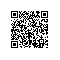# C++程序设计：原理与实践（进阶篇）16.1　标准库算法

Programming: Principles and Practice Using C++, Second Edition

——Trygve Reenskaug

16.1　标准库算法

r = f?ind(b,e,v) r指向[b:e)中v首次出现的位置

r = f?ind_if(b,e,p) r指向[b:e)中令p(x)为true的第一个元素x

x = count(b,e,v) x为v在[b:e)中出现的次数

x = count_if(b,e,p) x为[b:e)中满足p(x)为true的元素的个数

sort(b,e) 用<运算符对[b:e)排序

sort(b,e,p) 用谓词p对[b:e)排序

copy(b,e,b2) 将[b:e)拷贝至[b2:b2+(e-b))；b2之后应有足够的空间用于存储元素

unique_copy(b,e,b2) 将[b:e)拷贝至[b2:b2+(e-b))；不拷贝相邻的重复元素

merge(b,e,b2,e2,r) 将有序序列[b2:e2)和[b:e)合并，并放入[r:r+(e-b)+(e2-b2))之中

r = equal_range(b,e,v) r是有序范围[b:e)的一个子序列，且其中所有元素值均为v，本质上是通过二分搜索查找v

equal(b,e,b2) [b:e)和[b2:b2+(e-b))的所有元素对应相等？

x = accumulate(b,e,i) x是将i与[b:e]中所有元素进行累加的结果

x = accumulate(b,e,i,op) 与accumulate类似，但用op进行“求和”运算

x = inner_product(b,e,b2,i) x是[b:e)与[b2:b2+(e-b))的内积

x = inner_product(b,e,b2,i,op,op2) 与inner_product类似，但用op和op2取代内积的+和*